# How to filter an indicator fed by Arcade Expression

199
8
3 weeks ago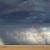New Contributor II

I am trying to get the "Damage Category Total" indicator to filter based off of the selection of a county. The gist is that respondents from across the state submit survey data on damages within their county, so I want to filter the results  by county. I know how to do it generally, and it works for the other Indicators, but when I filter by county, the "Damage Category Total" Indicator shows no data.

Here is a screenshot of my Dashboard in progress: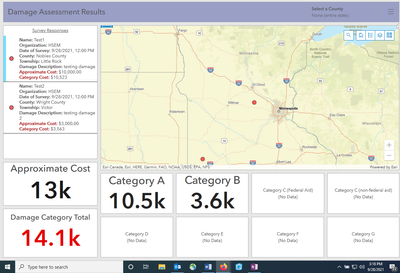The Indicators for the damage categories just display a sum of that field. The "Damage Category Total" Indicator requires an arcade expression since I am adding together the separate damage category fields.

Here is the expression:

``````var fs = FeatureSetByPortalItem(Portal('https://'),
'',0,
["emergency_cost_debris","emergency_cost_epm",
"permanent_cost_rb_fa","permanent_cost_rb_nfa",
"permanent_cost_wcf","permanent_cost_pbe",
"permanent_cost_pu", "permanent_cost_prf"],false);

var CategoryTotal= Sum(fs, "emergency_cost_debris") +
Sum (fs,"emergency_cost_epm") +
Sum (fs, "permanent_cost_rb_fa") +
Sum (fs, "permanent_cost_rb_nfa") +
Sum (fs, "permanent_cost_wcf")+
Sum (fs, "permanent_cost_pbe" )+
Sum (fs, "permanent_cost_pu" ) +
Sum (fs, "permanent_cost_prf" );

var newDict = {
'fields': [{ 'name': 'catTotal', 'type': 'esriFieldTypeDouble'}],
'geometryType':'',
'features': [{
'attributes': {
'catTotal': CategoryTotal,
}}]
};

return FeatureSet(Text(newDict));``````

Anybody know what I am missing? Each damage category filters appropriately based off the county picked (only displays the data collected within the selected county). Why would it not work for the arcade expression that is just a sum of those individual damage categories?

Thank you

1 Solution

Accepted Solutions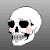byMVP Regular Contributor

Ah, I see. It looks like it's having trouble with the null values. Would it be inaccurate for your survey to default all answers to 0? Try updating the null values to 0 and then run the expression again.

If those aren't options, you can get this to work, but you've got to address those null values.

Instead of just invoking the field name, you'll need to use a case when... then... else... end within the sum_exp. Since GroupBy is submitting an SQL statement to the service, this all works.

`case when emergency_cost_debris is not null then emergency_cost_debris else 0 end +case when emergency_cost_epm is not null then emergency_cost_epm else 0 end`

But that would be a lot of typing, and a very long expression. One way to shorten this is to use a custom function.

``````// Auxiliary function for assembling expression
function field_exp(field) {
return `case when \${field} is not null then \${field} else 0 end`
};``````

This function will take the given string field and return the appropriate SQL statement. Using this, the expression above can be re-written as

``\${field_exp('emergency_cost_debris')} + \${field_exp('emergency_cost_epm')``

But even then, that would still end up  being quite a long expression. We can do one better by using a loop with a list of field names.

``````// List of fields to sum
var sum_fields = [
"emergency_cost_debris",
"emergency_cost_epm",
"permanent_cost_rb_fa",
"permanent_cost_rb_nfa",
"permanent_cost_wcf",
"permanent_cost_pbe",
"permanent_cost_pu",
"permanent_cost_prf"
]

// Instantiate sum_exp as empty string
var sum_exp = ''

for (var f in sum_fields) {
sum_exp += `\${field_exp(sum_fields[f])} + `
}``````

This results in the sum_exp value

`case when emergency_cost_debris is not null then emergency_cost_debris else 0 end + case when emergency_cost_epm is not null then emergency_cost_epm else 0 end + case when permanent_cost_rb_fa is not null then permanent_cost_rb_fa else 0 end + case when permanent_cost_rb_nfa is not null then permanent_cost_rb_nfa else 0 end + case when permanent_cost_wcf is not null then permanent_cost_wcf else 0 end + case when permanent_cost_pbe is not null then permanent_cost_pbe else 0 end + case when permanent_cost_pu is not null then permanent_cost_pu else 0 end + case when permanent_cost_prf is not null then permanent_cost_prf else 0 end + case when location_county is not null then location_county else 0 end + `

Nearly right, but there's the pesky " + " on the final item. Arcade doesn't have a nice "join" function like Python, but we can just trim it off like so.

`sum_exp = Left(sum_exp, Count(sum_exp) -3)`

An added benefit of this method is that the sum_fields array can be used as a parameter when requesting our FeatureSet. We just need to use Push() to add the county location field prior to the function.

### All Together Now!

``````// List of fields to sum
var sum_fields = [
"emergency_cost_debris",
"emergency_cost_epm",
"permanent_cost_rb_fa",
"permanent_cost_rb_nfa",
"permanent_cost_wcf",
"permanent_cost_pbe",
"permanent_cost_pu",
"permanent_cost_prf"
]

// Auxiliary function for assembling expression
function field_exp(field) {
return `case when \${field} is not null then \${field} else 0 end`
};

// Instantiate sum_exp as empty string
var sum_exp = ''

// Populate expression
for (var f in sum_fields) {
sum_exp += `\${field_exp(sum_fields[f])} + `
}

// Trim off last 3 characters
sum_exp = Left(sum_exp, Count(sum_exp) - 3)

// Push county field into fields array
Push(sum_fields, 'location_county')

// Get featureset
var fs = FeatureSetByPortalItem(
Portal('https://arcgis.com'),
'213a9271b441439196855a540ea797bb',
0,
sum_fields,
false);

// Group features by county
var g_fs = GroupBy(
fs,
'location_county',
[  // statistics to return for each county
{ name: 'Total', expression: '1', statistic: 'COUNT' },
{ name: 'catTotal', expression: sum_exp, statistic: 'SUM' }
]
);

return g_fs``````

Which returns:### The Indicator

Now you have your FeatureSet. The indicator can be set to do the full sum: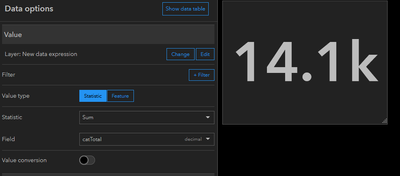Then on a category selector, list widget, etc., you can set a Filter action: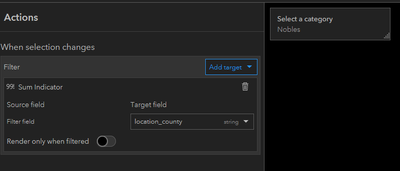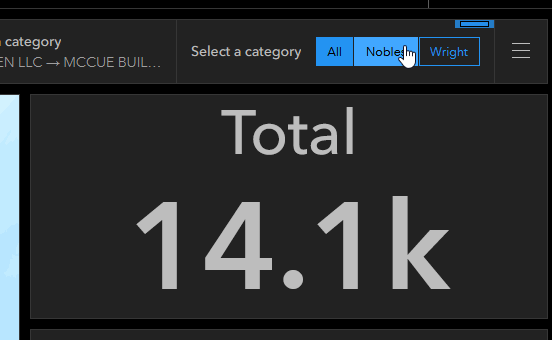It's alive... it's ALIVE!

- Josh Carlson
Kendall County GIS
8 RepliesbyMVP Regular Contributor

What you're missing is a "county" field that your output FeatureSet can filter by.

Assuming the 'county' field is available in that source layer, what you can do instead of your current expression is to use GroupBy.

``````var sum_exp = emergency_cost_debris + emergency_cost_epm + permanent_cost_rb_fa + permanent_cost_rb_nfa" + permanent_cost_wcf + permanent_cost_pbe + permanent_cost_pu + permanent_cost_prf

GroupBy(fs,
'county',
[  // statistics to return for each county
{ name: 'Total', expression: '1', statistic: 'COUNT' },
{ name: 'catTotal', expression: sum_exp, statistic: 'SUM' }
]
);``````

This will give you a FeatureSet with a single row per county, along with the number of features being summed and the total for all the fields.

In the indicator settings, you can set it to display the overall sum of these rows, achieving the same result as your initial expression, but now the FeatureSet can be acted upon based on its value in the county field.

- Josh Carlson
Kendall County GISNew Contributor II

Thanks for the reply Josh! I am new to Arcade, the expression I posted was hobbled together. What parts of it are you suggesting I replace?

Seems like I am replacing

`var CategoryTotal= Sum(fs, "emergency_cost_debris") + `

with

`var sum_exp = emergency_cost_debris + emergency_cost_epm + permanent_cost_rb_fa + permanent_cost_rb_nfa" + permanent_cost_wcf + permanent_cost_pbe + permanent_cost_pu + permanent_cost_prf`

and

```var newDict = {
'fields': [{ 'name': 'catTotal', 'type': 'esriFieldTypeDouble'}],
'geometryType':'',
'features': [{
'attributes': {
'catTotal': CategoryTotal,
}}]
}; ```

with

```GroupBy(fs,
'county',
[  // statistics to return for each county
{ name: 'Total', expression: '1', statistic: 'COUNT' },
{ name: 'catTotal', expression: sum_exp, statistic: 'SUM' }
]
);```

Is this what you are thinking? If so, is the var=sum_exp line how you actually add the different categories or do I still need to do it how I have it above, with

`Sum(fs, "emergency_cost_debris")`

for each category?

Tried a couple different things, I am getting Execution Error: h.charAt is not a function. I have added location_county to the fs at the top of the expression.byMVP Regular Contributor
``````// Get featureset
var fs = FeatureSetByPortalItem(
Portal('https://'),
'',
0,
[
"emergency_cost_debris",
"emergency_cost_epm",
"permanent_cost_rb_fa",
"permanent_cost_rb_nfa",
"permanent_cost_wcf",
"permanent_cost_pbe",
"permanent_cost_pu",
"permanent_cost_prf",
"location_county"
],
false);

// Establish sum expression
var sum_exp = "emergency_cost_debris + emergency_cost_epm + permanent_cost_rb_fa + permanent_cost_rb_nfa + permanent_cost_wcf + permanent_cost_pbe + permanent_cost_pu + permanent_cost_prf"

// Group features by county
var g_fs = GroupBy(
fs,
'location_county',
[  // statistics to return for each county
{ name: 'Total', expression: '1', statistic: 'COUNT' },
{ name: 'catTotal', expression: sum_exp, statistic: 'SUM' }
]
);

return g_fs``````

You've got it about right, the sum expression is replacing the per-category sum functions. What the GroupBy function is doing with that expression is for each row in the table, it will add up all of those fields' values, and will then sum those values for each unique value in "location_county".

Also, my mistake, that sum_exp var needs to be a string.

The above code should be everything you need, fingers crossed.

- Josh Carlson
Kendall County GISNew Contributor II

Ah, need to make the GroupBy a variable. Nice. It sort of works, except it does not actually sum the different categories. I previously could never get the sum expression you posted above to work. Do you think it matters if the fs I set includes a non numeric field (i.e. county)? I'm guessing it does for the way I was adding together the categories previously.

I'll pick this back up tomorrow, thank you so much for the help so far!byMVP Regular Contributor

No problem! Any chance the layer you're working with is public? If I could test the expression against the actual data, it would be that much more useful.

- Josh Carlson
Kendall County GISNew Contributor II

yeah for sure! Here is the link:

https://services2.arcgis.com/V12PKGiMAH7dktkU/arcgis/rest/services/service_0c81f2fdd9154719a45f33415...

Can also search for "PDA Survey Camp Ripley"byMVP Regular Contributor

Ah, I see. It looks like it's having trouble with the null values. Would it be inaccurate for your survey to default all answers to 0? Try updating the null values to 0 and then run the expression again.

If those aren't options, you can get this to work, but you've got to address those null values.

Instead of just invoking the field name, you'll need to use a case when... then... else... end within the sum_exp. Since GroupBy is submitting an SQL statement to the service, this all works.

`case when emergency_cost_debris is not null then emergency_cost_debris else 0 end +case when emergency_cost_epm is not null then emergency_cost_epm else 0 end`

But that would be a lot of typing, and a very long expression. One way to shorten this is to use a custom function.

``````// Auxiliary function for assembling expression
function field_exp(field) {
return `case when \${field} is not null then \${field} else 0 end`
};``````

This function will take the given string field and return the appropriate SQL statement. Using this, the expression above can be re-written as

``\${field_exp('emergency_cost_debris')} + \${field_exp('emergency_cost_epm')``

But even then, that would still end up  being quite a long expression. We can do one better by using a loop with a list of field names.

``````// List of fields to sum
var sum_fields = [
"emergency_cost_debris",
"emergency_cost_epm",
"permanent_cost_rb_fa",
"permanent_cost_rb_nfa",
"permanent_cost_wcf",
"permanent_cost_pbe",
"permanent_cost_pu",
"permanent_cost_prf"
]

// Instantiate sum_exp as empty string
var sum_exp = ''

for (var f in sum_fields) {
sum_exp += `\${field_exp(sum_fields[f])} + `
}``````

This results in the sum_exp value

`case when emergency_cost_debris is not null then emergency_cost_debris else 0 end + case when emergency_cost_epm is not null then emergency_cost_epm else 0 end + case when permanent_cost_rb_fa is not null then permanent_cost_rb_fa else 0 end + case when permanent_cost_rb_nfa is not null then permanent_cost_rb_nfa else 0 end + case when permanent_cost_wcf is not null then permanent_cost_wcf else 0 end + case when permanent_cost_pbe is not null then permanent_cost_pbe else 0 end + case when permanent_cost_pu is not null then permanent_cost_pu else 0 end + case when permanent_cost_prf is not null then permanent_cost_prf else 0 end + case when location_county is not null then location_county else 0 end + `

Nearly right, but there's the pesky " + " on the final item. Arcade doesn't have a nice "join" function like Python, but we can just trim it off like so.

`sum_exp = Left(sum_exp, Count(sum_exp) -3)`

An added benefit of this method is that the sum_fields array can be used as a parameter when requesting our FeatureSet. We just need to use Push() to add the county location field prior to the function.

### All Together Now!

``````// List of fields to sum
var sum_fields = [
"emergency_cost_debris",
"emergency_cost_epm",
"permanent_cost_rb_fa",
"permanent_cost_rb_nfa",
"permanent_cost_wcf",
"permanent_cost_pbe",
"permanent_cost_pu",
"permanent_cost_prf"
]

// Auxiliary function for assembling expression
function field_exp(field) {
return `case when \${field} is not null then \${field} else 0 end`
};

// Instantiate sum_exp as empty string
var sum_exp = ''

// Populate expression
for (var f in sum_fields) {
sum_exp += `\${field_exp(sum_fields[f])} + `
}

// Trim off last 3 characters
sum_exp = Left(sum_exp, Count(sum_exp) - 3)

// Push county field into fields array
Push(sum_fields, 'location_county')

// Get featureset
var fs = FeatureSetByPortalItem(
Portal('https://arcgis.com'),
'213a9271b441439196855a540ea797bb',
0,
sum_fields,
false);

// Group features by county
var g_fs = GroupBy(
fs,
'location_county',
[  // statistics to return for each county
{ name: 'Total', expression: '1', statistic: 'COUNT' },
{ name: 'catTotal', expression: sum_exp, statistic: 'SUM' }
]
);

return g_fs``````

Which returns:### The Indicator

Now you have your FeatureSet. The indicator can be set to do the full sum:Then on a category selector, list widget, etc., you can set a Filter action:It's alive... it's ALIVE!

- Josh Carlson
Kendall County GISNew Contributor II

You crushed it! I am amazed! There is literally no way I would have figured that out. I am going to spend some time reading over your answer, this will be really good practice for me.

Interesting part, I already had the questions in the survey set to 0 for the default!

Thank you so much! Wizard!